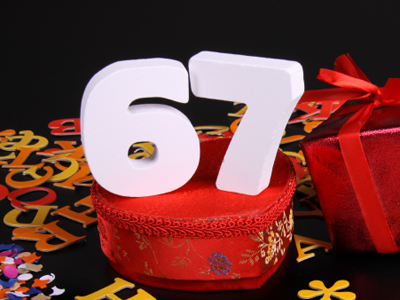The best method of multiplying 67 by 50 is to multiply by 100 then halve.

# Multiplication and Division 3

This Math quiz is called 'Multiplication and Division 3' and it has been written by teachers to help you if you are studying the subject at elementary school. Playing educational quizzes is an enjoyable way to learn if you are in the 3rd, 4th or 5th grade - aged 8 to 11.

It costs only \$12.50 per month to play this quiz and over 3,500 others that help you with your school work. You can subscribe on the page at Join Us

Multiplication and division involves splitting numbers into parts, or fractions (in the case of division). Some divisions are straightforward, such as dividing 20 bananas between 4 monkeys. Each monkey will get 5 bananas each. Other divisions are not so simple!

Multiplying and dividing can be quite hard with decimals and fractions, but once you know how to go about it it does get easier! To multiply a number by 14 you divide it by 4. The same is true for decimals: to multiply a number by 0.25 you also divide it by 4.

Test your knowledge of multiplication and division by trying the following quiz on the subject.

1.
3 + (5 x 8) =
(3 + 5) x 8 =
3 + 5 x 8 =
(3 + 8) x 5 =
The calculation in the brackets is always done first
2.
What is the best method of multiplying 67 by 50?
Multiply by 100 then halve
Multiply by 100 then double
Multiply by 5 then multiply by 10
Divide by 100 then double
100 and 2 are easier numbers to multiply and divide than 50 is
3.
How do we find a third of a number?
Multiply it by 3
Divide it by 6
Multiply it by 6
Divide it by 3
A third means the same as ÷ 3
4.
22 ÷ 4 = 5 r 2. What fraction is the remainder 2?
Half
Quarter
Third
Eighth
The remainder can be expressed as 24 or 12
5.
Which of these methods would result in finding 16?
Half one third
Half one quarter
Double one quarter
Double one third
A sixth is half a third
6.
On a calculator the answer to a division is 7.2. How is this interpreted in money?
£72
£7.2
£7.20
£0.72
720p is the same as £7.20
7.
How can one twentieth be calculated?
Find one tenth and halve
Find one fifth and double
Find one tenth and double
Find one fifth and halve
One twentieth is half of one tenth
8.
Which strategy would be best to multiply 79 by 21?
Multiply by 10 then subtract 79
Multiply by 20 then add 79
Multiply by 10 then add 79
Multiply by 20 then subtract 79
To simplify it further you could multiply 79 by 2, then by 10 and finally add 79
9.
Which strategy would be best to multiply 42 by 16?
Multiply by 8 then double
Multiply by 4 then double
Divide by 2 then multiply by 8
Divide by 8 then double
If a number in a problem is difficult to work with then try to break it down into easier to deal with numbers
10.
What is the best method of multiplying 58 by 9?
Multiply by 10 then add 58
Multiply by 5 then add 58
Multiply by 10 then subtract 58
Multiply by 5 then subtract 58
10 is a much easier number to multiply than 9 is
Author:  Amanda Swift